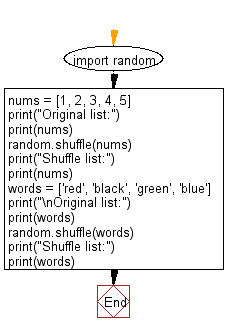﻿ Python: Shuffle the elements of a given list - w3resource# Python: Shuffle the elements of a given list

## Python module: Exercise-6 with Solution

Write a Python program to shuffle the elements of a given list.

Use random.shuffle()

Sample Solution:

Python Code:

``````import random
nums = [1, 2, 3, 4, 5]
print("Original list:")
print(nums)
random.shuffle(nums)
print("Shuffle list:")
print(nums)
words = ['red', 'black', 'green', 'blue']
print("\nOriginal list:")
print(words)
random.shuffle(words)
print("Shuffle list:")
print(words)
```
```

Sample Output:

```Original list:
[1, 2, 3, 4, 5]
Shuffle list:
[4, 2, 1, 5, 3]

Original list:
['red', 'black', 'green', 'blue']
Shuffle list:
['green', 'blue', 'black', 'red']
```

Flowchart:## Visualize Python code execution:

The following tool visualize what the computer is doing step-by-step as it executes the said program:

Python Code Editor:

Have another way to solve this solution? Contribute your code (and comments) through Disqus.

What is the difficulty level of this exercise?

Test your Python skills with w3resource's quiz

﻿

## Python: Tips of the Day

Dictionary comprehension:

```>>> m = {x: x ** 2 for x in range(5)}
>>> m
{0: 0, 1: 1, 2: 4, 3: 9, 4: 16}
```